# Limit of function at infinity

Definition: Let be a function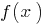defined on the entire number line. The numberis called the limit of the functionatif for any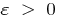there exists a number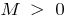that for allsatisfying the condition, the following inequality is satisfied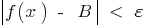If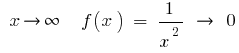, that is, for large (absolute) values ofthe number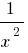very little different from the number 0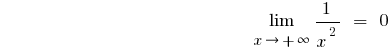If the behavioris different withandseparately consider(in the definition of take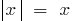) and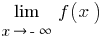(definition of take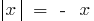)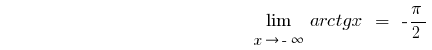## The limit of a sequence

Since a sequence is a function of natural argument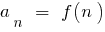, the definition of limit of a sequence withis identical to the definition of limit of a function atDefinition: a Numberis called the limit of a sequenceif for anythere is such numberthat for all, the following inequality is satisfied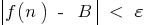i.e.If,## Comparison of exponential growth, exponential and logarithmic functions

• When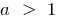•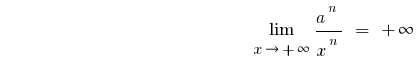,

that is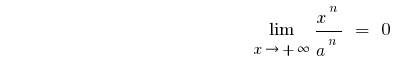If, when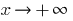a function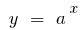grows faster than any exponential function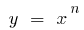where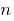is a natural number

Graphically, this statement means that for sufficiently large values ofthe graph of the function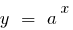(where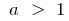) is above the graph of a function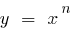• When•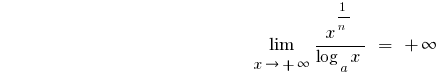,

that is,

At large;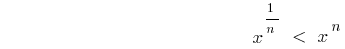,

soIf, the function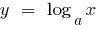increases slower than the function(and especially slower than a function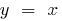or a function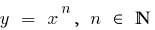)

Graphically, this statement means that for sufficiently large values ofthe graph of the function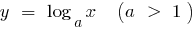lies below the graph of a function(and especially below the graphs of functions)

Tags:
Chapter:
Versions in other languages:
Share with friends: